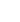15min:
CHIRAL CAVITY RING-DOWN: ABSOLUTE MEASUREMENT OF OPTICAL ROTATION IN GASES AND LIQUIDS WITH SIGNAL REVERSALS.

LYKOURGOS BOUGAS, G. E. KATSOPRINAKIS, T. P. RAKITZIS, Department of Physics, University of Crete, and Institute of Electronic Structure and Laser, Foundation for Research and Technology-Hellas 71110 Heraklion-Crete, Greece.

We demonstrate the absolute measurement of optical rotation using a four-mirror cavity-ring-down polarimetric setup. A four-mirror bow-tie cavity allows counter-propagating laser beams, for which symmetry is broken with a longitudinal magnetic field acting upon an intracavity magneto-optic window (producing a Faraday rotation_F). A chiral sample is introduced in one arm of the cavity, producing a chiral rotation__C. The different symmetry of the two rotations produces a total rotation of (_F +__C) for one laser beam, and (_F +__C) for the counter-propagating beam. These rotations produce a polarization beating in the cavity ring-down, of frequency__CW and__CCW for the clockwise and counter-clockwise beams, respectively. Analysis of the difference (__CW-__CCW) yields the chiral rotation angle \pm__C, where the sign of the angle is determined by the sign of the applied magnetic field. Therefore, subtracting the signals using +\vecB and Đ\vecB yields 2__C, allowing the absolute determination of__C, without needing to remove the sample . We demonstrate these absolute optical rotation measurements for chiral gases (-pinene) and chiral liquids.
This work is a proof-of-principle demonstration of the experimental setup proposed for the measurement of parity non-conserving optical rotation in atomic systems .

\beginitemize em L. Bougas, G. E. Katsoprinakis, W. von Klitzing, J. Sapirstein, and T. P. Rakitzis, Phys. Rev. Lett 108, 210801 (2012). \enditemize